# Civil Engineering - UPSC Civil Service Exam Questions

16.

If 'p' is the precipitation, 'a' is the area represented by a rainguage, and n is the number of raingauges in a catchment area, then the weighted mean rainfall is

 A.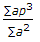B.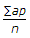C.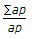D.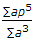Explanation:

No answer description available for this question. Let us discuss.

17.

For the beam shown in the given figure, the maximum positive bending moment is equal to negative bending moment. The value of L1 is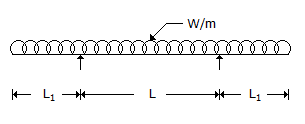A.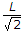B.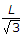C.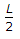D.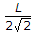Explanation:

No answer description available for this question. Let us discuss.

18.

A plane pin-jointed truss is shown in the figure. The force resisted by the member AD is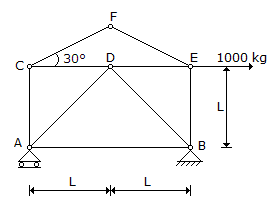A. zero B. 5002 kg. (compression) C. 5002 kg. (tension) D. 10002 kg. (tension)

Explanation:

No answer description available for this question. Let us discuss.

19.

In the figure shown aside, which one of the following correctly represents effective stress at C ? (Symbols have the usual meanings).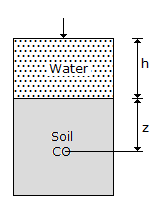A. (h + z)γω B. z.γω C. z.γsub D. h.γω + Z.γsat

Explanation:

No answer description available for this question. Let us discuss.

20.

What is range of values of Poisson's ratio for ductile materials ?

 A. 0.10 to 0.15 B. 0.16 to 0.20 C. 0.21 to 0.24 D. 0.25 to 0.33